# Poisson transform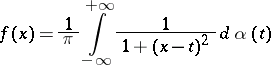(*)

whereis a function of bounded variation in every finite interval, and also the transform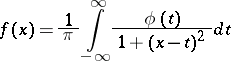which results from (*) ifis an absolutely-continuous function (cf. Absolute continuity). Letand letThe following inversion formulas hold for the Poisson transform: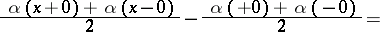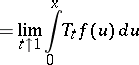for all, and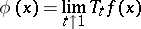almost everywhere.

Letbe a convex open acute cone inwith vertex at zero and letbe the dual cone, that is,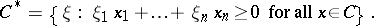The function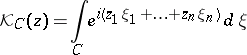is called the Cauchy kernel of the tube domain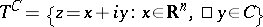. The Poisson transform of a (generalized) functionis the convolution (cf. Convolution of functions)where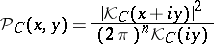is the Poisson kernel of the tube domain(see ).